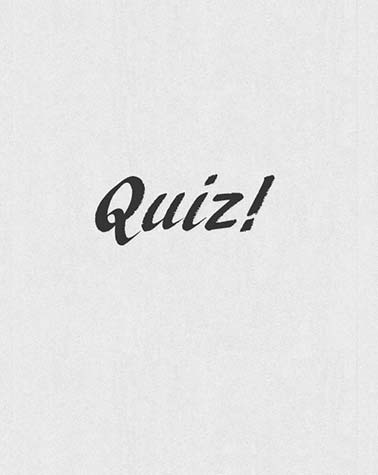# Measuring With Standard And Metric Measurements

Approved & Edited by ProProfs Editorial Team
The editorial team at ProProfs Quizzes consists of a select group of subject experts, trivia writers, and quiz masters who have authored over 10,000 quizzes taken by more than 100 million users. This team includes our in-house seasoned quiz moderators and subject matter experts. Our editorial experts, spread across the world, are rigorously trained using our comprehensive guidelines to ensure that you receive the highest quality quizzes.
| By Princesstiffany2
P
Princesstiffany2
Community Contributor
Quizzes Created: 1 | Total Attempts: 243
Questions: 10 | Attempts: 243SettingsMrs. Bolding's Pre-Engineering/STEM Class

• 1.

### How many inches are in a foot?

• A.

10 Inches

• B.

12 Inches

• C.

6 Inches

• D.

20 Inches

B. 12 Inches
Explanation
There are 12 inches in a foot. This is a standard conversion in the Imperial system of measurement.

Rate this question:

• 2.

### How many inches are in a yard?

• A.

12 Inches

• B.

30 Inches

• C.

36 Inches

• D.

40 Inches

C. 36 Inches
Explanation
There are 36 inches in a yard. This is a standard conversion in the Imperial system. Since there are 12 inches in a foot and 3 feet in a yard, multiplying 12 by 3 gives us the answer of 36 inches in a yard.

Rate this question:

• 3.

### How many feet are in a yard?

• A.

3 Feet

• B.

4 Feet

• C.

2 Feet

• D.

8 Feet

A. 3 Feet
Explanation
There are 3 feet in a yard. This is a standard measurement conversion in the imperial system. A yard is a unit of length equal to 3 feet, so when converting from yards to feet, you multiply the number of yards by 3.

Rate this question:

• 4.

### What units would you use to measure with English/Standard?

• A.

Millimeters

• B.

Inches

• C.

Centimeters

• D.

Elephants

B. Inches
Explanation
Inches would be the correct unit to measure with English/Standard. Inches are commonly used in countries that follow the English/Standard system of measurement. Millimeters and centimeters are units of measurement in the metric system, which is not used in English/Standard. Elephants is not a unit of measurement and therefore not a valid option.

Rate this question:

• 5.

### What units would you use to measure with Metric Measurements?

• A.

Yards

• B.

Inches

• C.

Camel Feet

• D.

Millimeters

D. Millimeters
Explanation
Metric measurements are based on the metric system, which is a decimal-based system of measurement. The units in the metric system are all based on powers of ten, making conversions between units easy. Millimeters are a unit of length in the metric system, and they are commonly used for small measurements, such as the thickness of a sheet of paper or the diameter of a pencil. Yards, inches, and camel feet are not units of measurement in the metric system.

Rate this question:

• 6.

### How many millimeters are in a centimeter?

• A.

8

• B.

10

• C.

12

• D.

A bunch

B. 10
Explanation
There are 10 millimeters in a centimeter. This is a standard conversion in the metric system, where 1 centimeter is equal to 10 millimeters. Therefore, the correct answer is 10.

Rate this question:

• 7.

### How many equal parts can an inch be broken into?

• A.

16

• B.

12

• C.

14

• D.

Too many

A. 16
Explanation
An inch can be broken into 16 equal parts because there are 16 marks on a standard ruler, each representing 1/16th of an inch.

Rate this question:

• 8.

### 12ft 6 in= __________________ inches

• A.

126 inches

• B.

150 inches

• C.

140 inches

• D.

Well 12+6 is 18, so 18 inches

B. 150 inches
Explanation
The correct answer is 150 inches. This is because 12ft 6in can be converted to inches by multiplying 12 by 12 (to convert feet to inches) and adding 6 inches. So, 12ft 6in is equal to 144 inches + 6 inches, which equals 150 inches.

Rate this question:

• 9.

### 119ft= ___________yd __________ft

• A.

30 yards 4 feet

• B.

110 yards 9 feet

• C.

32 yards 2 feet

• D.

I mow 1 yard and have 2 feet so 1 yard 2 feet

C. 32 yards 2 feet
Explanation
The given answer, 32 yards 2 feet, is the correct conversion of 119 feet into yards and feet. Since there are 3 feet in a yard, we can divide 119 by 3 to get the number of yards, which is 39 yards with a remainder of 2 feet. Therefore, the answer is 32 yards 2 feet.

Rate this question:

• 10.

### 2.39 cm= ___________mm

• A.

23.9

• B.

239

• C.

0.239

• D.

Wait what? Why is there a decimal?Back to top